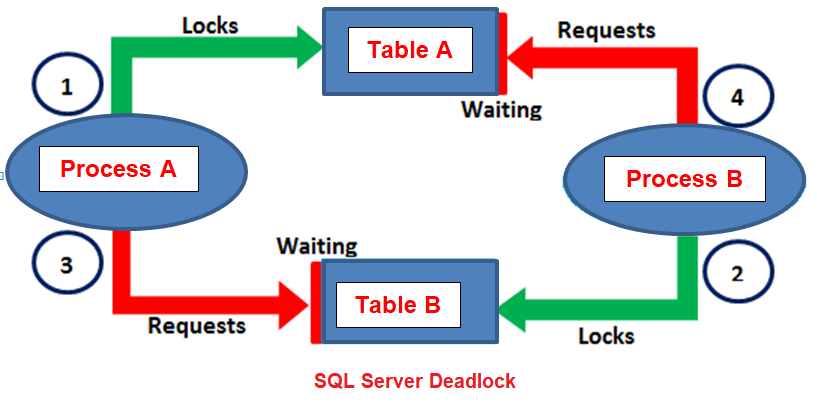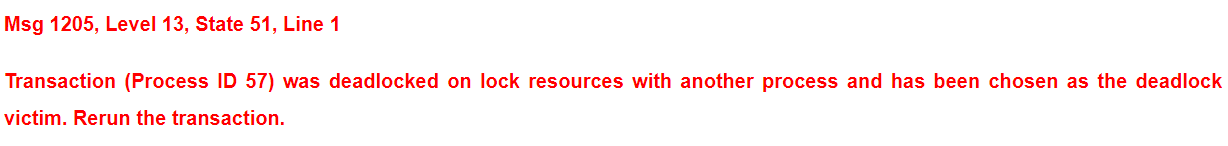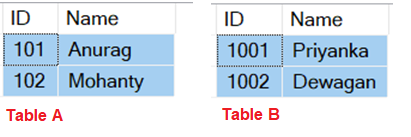## Deadlock in SQL Server with Examples

In this article, I am going to discuss Deadlock in SQL Server with example detail. Please read our previous article where as discussed Concurrency in SQL Server with examples. Before going to understand what is Deadlock in SQL Server, let’s first discuss when a deadlock occurs in SQL Server.

##### When a deadlock occurs in SQL Server?

A deadlock occurs in a database when two or more processes have already a resource locked, and then each process wants to acquire a lock on the resource that the other process has already locked. In such cases, neither of the processes can move forward, as each process is waiting for the other process to release the lock.

If you are confused, then just have a look at the following diagram which explains the above points i.e. when a deadlock occurs in a database.As shown in the above diagram, we have two processes (process means transactions) such as Process A and Process B. We have two tables such as Table A and Table B in the database. The Process A started and it acquires a lock on table A and in the meantime the Process B started and it acquired a lock on Table B. Now to complete its execution, the process A needs the resource Table B which is already locked by the Process B. In the same the Process B needs the resource Table A which is already locked by the Process A. In such cases neither of the transactions (processes) can move forward resulting a deadlock.

When deadlocks occur in SQL Server, then the SQL Server chooses one of the processes (transactions) as the deadlock victim and then rollback that process. As a result, other processes can move forward. The process that is chosen as the deadlock victim will give the following error.##### Let us understand Deadlock with an example.

We are going to use the following two tables in this demo.Please use the below SQL Script to create and populate the tables with the test data.

```-- Create table TableA
CREATE TABLE TableA
(
ID INT,
Name NVARCHAR(50)
)
Go

-- Insert some test data
INSERT INTO TableA values (101, 'Anurag')
INSERT INTO TableA values (102, 'Mohanty')
Go

-- Create table TableB
CREATE TABLE TableB
(
ID INT,
Name NVARCHAR(50)
)
Go

-- Insert some test data
INSERT INTO TableB values (1001, 'Priyanka')
INSERT INTO TableB values (1002, 'Dewagan')
Go
```

The following 2 transactions will result in a deadlock situation. Open 2 instances of SQL Server Management Studio. From the first instance execute Transaction 1 code and from the second instance execute Transaction 2 code.

###### Transaction 1 Code:
```-- Transaction 1
-- Step1 Start
BEGIN TRANSACTION
UPDATE TableA Set Name = 'Anurag From Transaction1' WHERE Id = 101
-- Step1 End

-- From Transaction 2 Window execute the step2 section

-- Step3 Start
UPDATE Tableb Set Name = 'Priyanka From Transaction1' WHERE Id = 1001
-- Step3 End

-- From Transaction 2 Window execute the step4 section
COMMIT TRANSACTION
```
###### Transaction 2 Code:
```-- Transaction 2
-- Step2 Start
BEGIN TRANSACTION
UPDATE TableB Set Name = 'Priyanka From Transaction2' WHERE Id = 1001
-- Step2 End

-- From Transaction 1 window execute the Step3 section
UPDATE TableA Set Name = 'Anurag From Transaction2' WHERE Id = 101

Commit Transaction
```

You will notice that after a few seconds one of the transactions completed successfully while the other transaction is chosen as the deadlock victim by giving the following error.